# Text Function

6.1) CHAR:  - This function required number between 1 to 255 specifying which character you want. The character is from the character set used by your computer

Syntax:  =

Example6.2) CODE: - This function returns the ASCII value of a character, this function return character number

Syntax: =

Example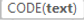6.3) UNICHAR: - This function give you the Unicode characters from the Unicode number provided.

Syntax: =

Example6.4) UNICODE: - This function give you Unicode of string/texts

Syntax: =

Example6.5) CLEAN: - Remove non-printable characters (Non-printable Unicode characters include No. 1429,141,143,144 & 157)

Syntax:  =

Example6.6) TEXT: - The easiest way to change number to text

Syntax: =

Example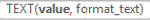6.7) NUMBERVALUE: - This function is use to change text value to number with decimal and group separators.

Syntax: =

Example6.8) FIXED: - This function apply decimal points with prevent commas or do not prevent commas to any number,

Syntax: =

Example6.9) DOLLAR: This function change the number to text with currency format with the decimals place round as per you specify.

Syntax: =

Example6.10) T: - This function check the text/string

Syntax: =

Example6.11) VALUE: - This function give you a numeric value

Syntax: =

Example6.12) LEN: - This function use to counts characters, letters and space in the cells (Count the length of text)

Syntax: =

Example6.13) LEFT: - This function is used to extract left side’s number of text

Syntax:=

Example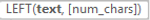6.14) RIGHT: - This function is used to extract right side’s number of text

Syntax: =

Example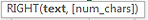6.15) MID: - Return the character of given number from middle of a supplied text string

Syntax: =

Example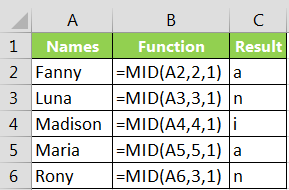6.16) REPT: - This function give you repetitive text as per specified number to times.

Syntax:=

Example6.17) EXACT: - This function used for check or compare both text are same or not, consider upper and lower case also (Result in True or False)

Syntax: =

Example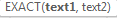6.18) CONCATENATE: - This function joint multiple character and values in on word

Syntax:=

Example6.19) TRIM: - This function remove unwanted starting and ending space

Syntax:=

Example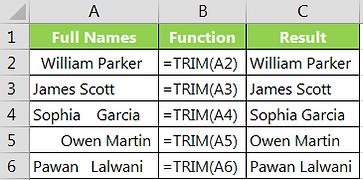6.20) LOWER: - change text case in lower case

Syntax: =

Example6.21) UPPER: This function is used to change text case in capital (upper case)

Syntax: =

Example6.22) PROPER: - This function is used to change text case in first letter in capital then small case.

Syntax: =

Example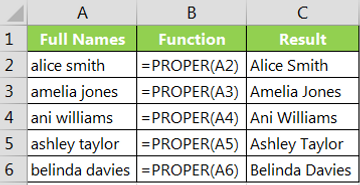6.23) FIND: - This function is use to find out text number within any word from starting position, consider upper & lower case also

Syntax: =

Example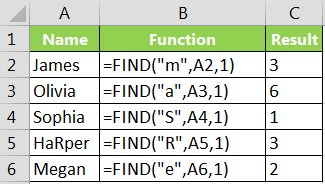6.24) REPLACE: - This function is used to replaces character specified by position in given text string with another text string

Syntax:=

Example =Replace (“Vikas123”, 6, 3,”456”)     Vikas4566.25) SEARCH: - This function returns a number value. The first position.

Syntax: =

Example6.26) SUBSTITUTE: - This function is replace a group of string with another string.

Syntax: =

Example